## Saturday, 9 August 2014

### chapter 3 - Worked Out Examples

 Example: 1
 Use logs to evaluate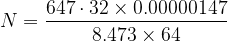$N = \dfrac{{647 \cdot 32 \times 0.00000147}}{{8.473 \times 64}}$
 Solution: 1
Our approach consists of four steps:
• – convert the expression for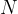$N$ into logs
• evaluate those logs using a logs – table
• thus determine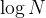$\log N$
• calculate antilog of$\log N$
Thus,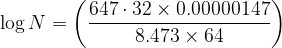$\log N = \left( {\dfrac{{647 \cdot 32 \times 0.00000147}}{{8.473 \times 64}}} \right)$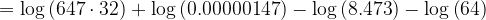$= \log \left( {647 \cdot 32} \right) + \log \left( {0.00000147} \right) - \log \left( {8.473} \right) - \log \left( {64} \right)$
Now, note that (use log tables):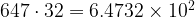$647 \cdot 32 = 6.4732 \times {10^2}$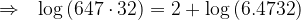$\Rightarrow \,\,\,\, \log \left( {647 \cdot 32} \right) = 2 + \log \left( {6.4732} \right)$$= 2 + \,\,\, \cdot 8111$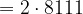$= 2 \cdot 8111$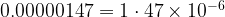$0.00000147 = 1 \cdot 47 \times {10^{ - 6}}$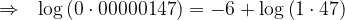$\Rightarrow \,\,\,\, \log \left( {0 \cdot 00000147} \right) = - 6 + \log \left( {1 \cdot 47} \right)$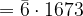$= \bar 6 \cdot 1673$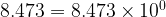$8.473 = 8.473 \times {10^0}$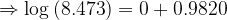$\Rightarrow \log \left( {8.473} \right) = 0 + 0.9820$$= 0.9820$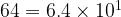$64 = 6.4 \times {10^1}$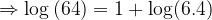$\Rightarrow \log \left( {64} \right) = 1 + \log (6.4)$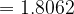$= 1.8062$
Thus,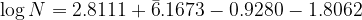$\log N = 2.8111 + \bar 6.1673 - 0.9280 - 1.8062$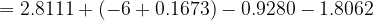$= 2.8111 + ( - 6 + 0.1673) - 0.9280 - 1.8062$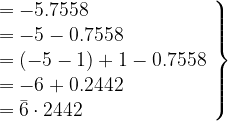$\left. \begin{array}{l} = - 5.7558\\ = - 5 - 0.7558\\ = \left( { - 5 - 1} \right) + 1 - 0.7558\\ = - 6 + 0.2442\\ = \bar 6 \cdot 2442 \end{array} \right\}$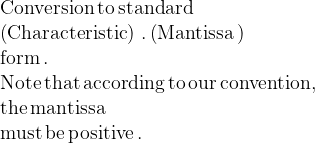$\begin{array}{l} {\rm{Conversion}}\,{\rm{to \,standard}}\\ \left( {{\rm{Characteristic}}} \right)\,.\left( {{\rm{Mantissa}}}\, \right)\\ {\rm{form}}\,{\rm{.}}\\ {\rm{Note\, that\, according\, to\, our \,convention,\,}}\\ {\rm{the \,mantissa\,}}\\ {\rm{must\, be\, positive\,}}{\rm{.}} \end{array}$
Now, we have the characteristic and mantissa of$\log N$.
We thus find antilog (mantissa)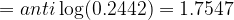$= anti\log (0.2442) = 1.7547$ {from your antilog tables}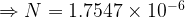$\Rightarrow N = 1.7547 \times {10^{ - 6}}$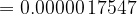$= 0.00000\,17547$
Verify this using a calculator. Note that$N$ was calculated without any actual multiplication and division – operations much more cumbersome than addition or subtraction.
 Example: 2
 Find the value of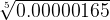$\sqrt{{0.00000165}}$
 Solution: 2
Let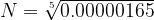$N = \sqrt{{0.00000165}}$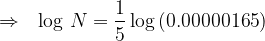$\Rightarrow \,\,\,\, \log \,N = \dfrac{1}{5}\log \left( {0.00000165} \right)$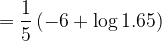$= \dfrac{1}{5}\left( { - 6 + \log 1.65} \right)$ (Why?)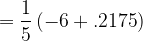$= \dfrac{1}{5}\left( { - 6 + .2175} \right)$$= - 1.2 + 0.0435$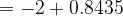$= - 2 + 0.8435$ Why did we do this?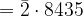$= \bar 2 \cdot 8435$
Thus,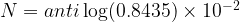$N = anti\log (0.8435) \times {10^{ - 2}}$$6.974 \times {10^{ - 2}}$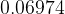$0.06974$